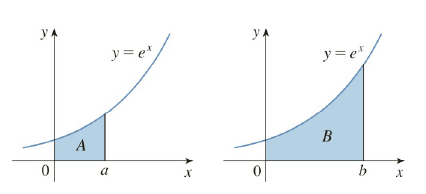Chapter 4.4, Problem 74E

Chapter
Section
Textbook Problem

# The area labeled B is three times the area labeled A. Express b in terms of a.To determine

To express:

b in terms of a.

Explanation

1) Concept:

Use the given condition to express b in terms of a

2) Calculation:

From the given graph,

Area bounded by the curve y=ex for region A is 0aexdx

Area bounded by the curve y=ex for region B is 0bexdx

Here,

the area labeled B is three times the area labeled A that is B=3A So

0bex dx=30aexdx

### Still sussing out bartleby?

Check out a sample textbook solution.

See a sample solution

#### The Solution to Your Study Problems

Bartleby provides explanations to thousands of textbook problems written by our experts, many with advanced degrees!

Get Started

#### Evaluate the expression sin Exercises 116. (14)2

Finite Mathematics and Applied Calculus (MindTap Course List)

#### Evaluate the integral. /6/2csctcottdt

Single Variable Calculus: Early Transcendentals, Volume I

#### In Problems 9-20, graph each function. 19.

Mathematical Applications for the Management, Life, and Social Sciences

#### Prove that limxxesin(/x)=0.

Single Variable Calculus: Early Transcendentals

#### If f(x) = 2e3x, f(x) = a) 2e3x b) 2xe3x c) 6e3x d) 6xe3x

Study Guide for Stewart's Single Variable Calculus: Early Transcendentals, 8th

#### Write a research report using APA style.

Research Methods for the Behavioral Sciences (MindTap Course List)